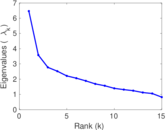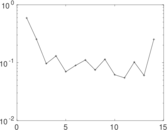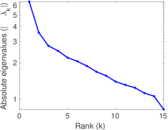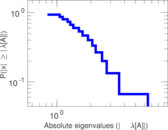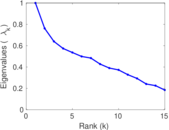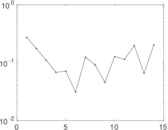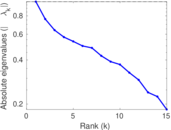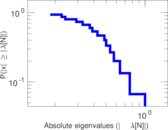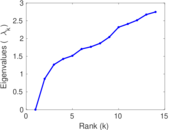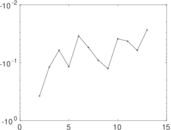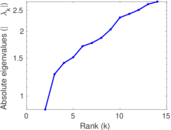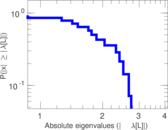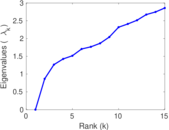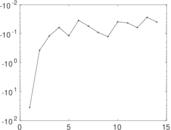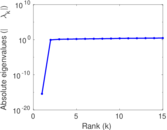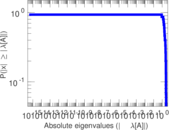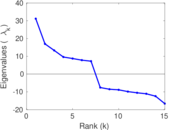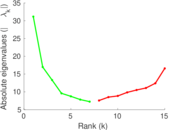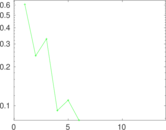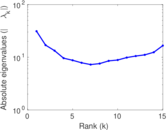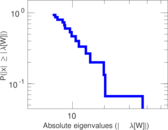# Corporate club memberships

This bipartite network contains membership information of corporate executive officers in social organizations such as clubs and boards. Left nodes represent persons and right nodes represent social organisations. An edge between a person and a social organization shows that the person is a member.

 Code `BM` Internal name `brunson_club-membership` Name Corporate club memberships Data source https://github.com/corybrunson/triadic AvailabilityDataset is available for download Consistency checkDataset passed all tests Category Affiliation network Node meaning Person, organization Edge meaning Membership Network formatBipartite, undirected Edge typeUnweighted, no multiple edges

## Statistics

 Size n = 40 Left size n1 = 25 Right size n2 = 15 Volume m = 95 Wedge count s = 534 Claw count z = 1,935 Cross count x = 6,874 Square count q = 212 4-Tour count T4 = 4,186 Maximum degree dmax = 21 Maximum left degree d1max = 7 Maximum right degree d2max = 21 Average degree d = 4.750 00 Average left degree d1 = 3.800 00 Average right degree d2 = 6.333 33 Fill p = 0.253 333 Size of LCC N = 40 Diameter δ = 5 50-Percentile effective diameter δ0.5 = 2.066 34 90-Percentile effective diameter δ0.9 = 3.373 17 Median distance δM = 3 Mean distance δm = 2.546 67 Gini coefficient G = 0.300 632 Balanced inequality ratio P = 0.384 211 Left balanced inequality ratio P1 = 0.421 053 Right balanced inequality ratio P2 = 0.347 368 Relative edge distribution entropy Her = 0.954 522 Power law exponent γ = 2.375 30 Tail power law exponent γt = 2.871 00 Tail power law exponent with p γ3 = 2.871 00 p-value p = 0.238 000 Left tail power law exponent with p γ3,1 = 8.031 00 Left p-value p1 = 0.927 000 Right tail power law exponent with p γ3,2 = 2.401 00 Right p-value p2 = 0.558 000 Degree assortativity ρ = −0.113 162 Degree assortativity p-value pρ = 0.274 887 Spectral norm α = 6.465 51 Algebraic connectivity a = 0.867 610 Spectral separation |λ1[A] / λ2[A]| = 1.806 22 Controllability C = 10 Relative controllability Cr = 0.250 000

## Plots

### Fruchterman–Reingold graph drawing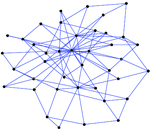### Degree distribution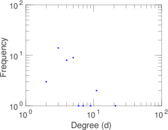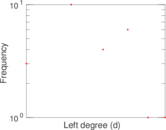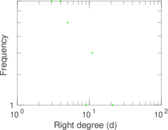### Cumulative degree distribution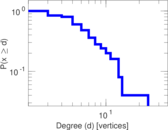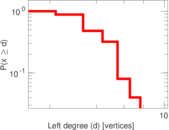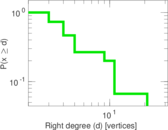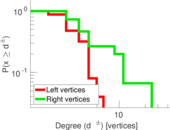### Lorenz curve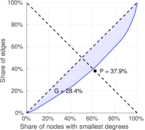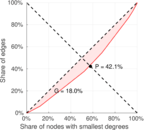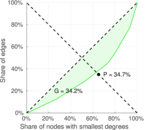### Spectral distribution of the adjacency matrix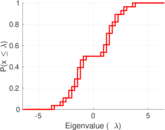### Spectral distribution of the normalized adjacency matrix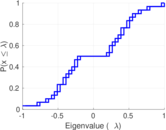### Spectral distribution of the Laplacian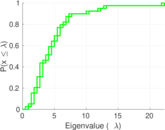### Spectral graph drawing based on the adjacency matrix### Spectral graph drawing based on the Laplacian### Spectral graph drawing based on the normalized adjacency matrix### Degree assortativity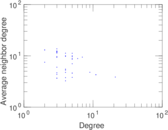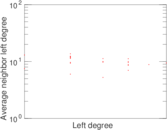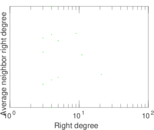### Zipf plot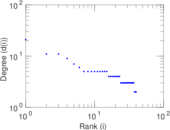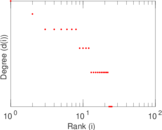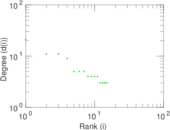### Hop distribution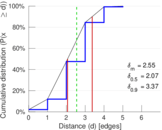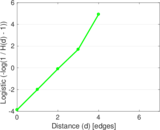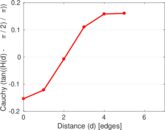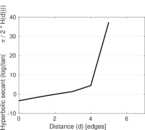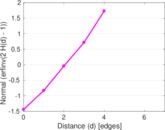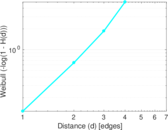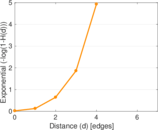### Double Laplacian graph drawing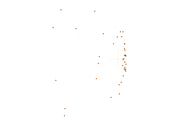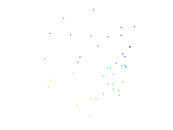### Delaunay graph drawing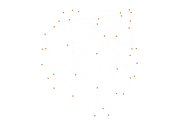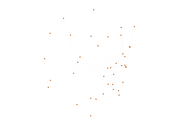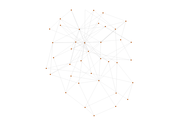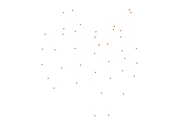### Matrix decompositions plots